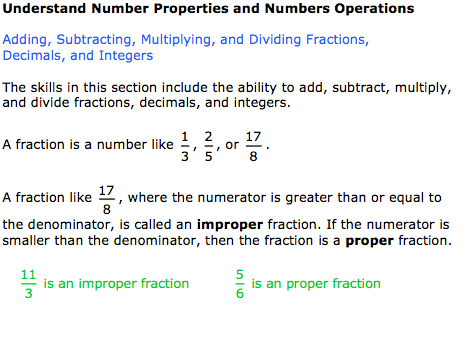Welcome, Student Name | Student Demo | Buy Now

 Contents Mathematics (190)Number Properties and OperationsFractions, Decimals, and IntegersRatios and ProportionsPercentsApplication Problems Using PercentsEvaluating ExponentsStandard Notation and Scientific NotationPrinciples of AlgebraMeasurement and Geometry ConceptsProbability and StatisticsProblem Solving and ReasoningPractice Math Tests Main Menu Exit Student Demo Buy Now for \$39.95 and begin using the entire course in less than two minutes